Скачать презентацию Chapter 4 Two-Dimensional Kinematics James Walker Physics 3

fec15923db754a1ffbe57741db4ac029.ppt

• Количество слайдов: 14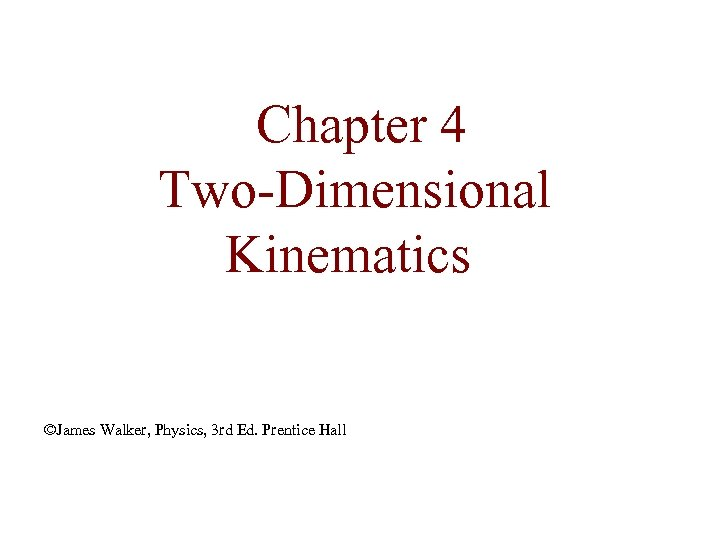Chapter 4 Two-Dimensional Kinematics ©James Walker, Physics, 3 rd Ed. Prentice Hall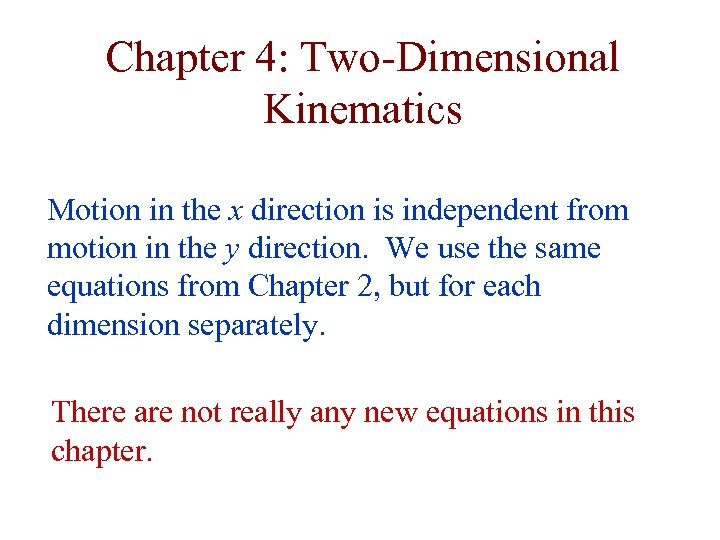Chapter 4: Two-Dimensional Kinematics Motion in the x direction is independent from motion in the y direction. We use the same equations from Chapter 2, but for each dimension separately. There are not really any new equations in this chapter.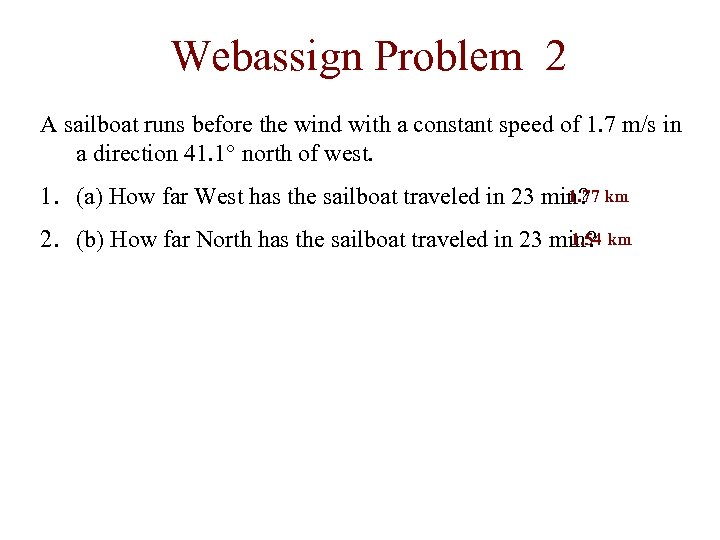Webassign Problem 2 A sailboat runs before the wind with a constant speed of 1. 7 m/s in a direction 41. 1° north of west. 1. 77 1. (a) How far West has the sailboat traveled in 23 min? km 1. 54 2. (b) How far North has the sailboat traveled in 23 min? km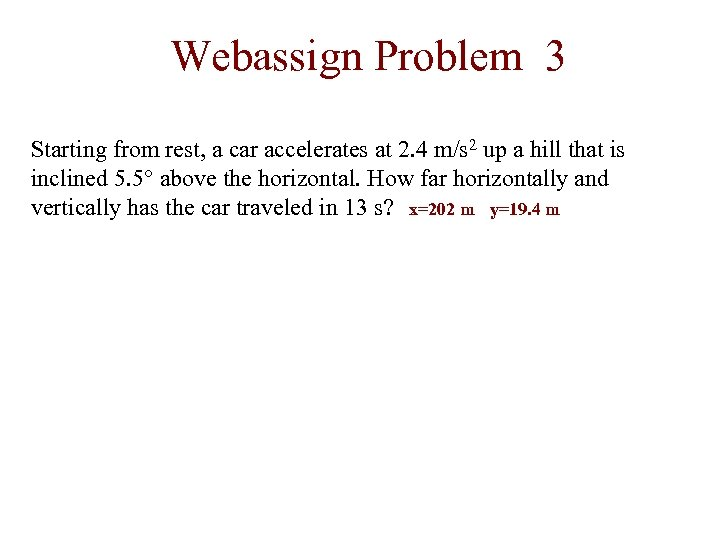Webassign Problem 3 Starting from rest, a car accelerates at 2. 4 m/s 2 up a hill that is inclined 5. 5° above the horizontal. How far horizontally and vertically has the car traveled in 13 s? x=202 m y=19. 4 m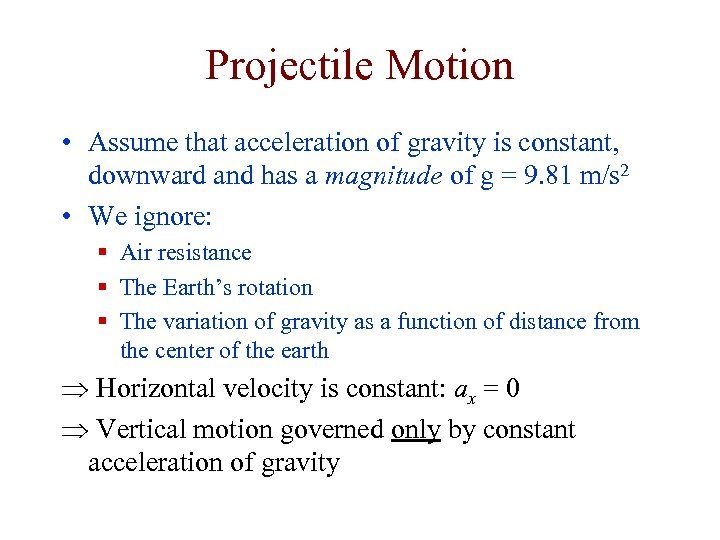Projectile Motion • Assume that acceleration of gravity is constant, downward and has a magnitude of g = 9. 81 m/s 2 • We ignore: § Air resistance § The Earth’s rotation § The variation of gravity as a function of distance from the center of the earth Þ Horizontal velocity is constant: ax = 0 Þ Vertical motion governed only by constant acceleration of gravity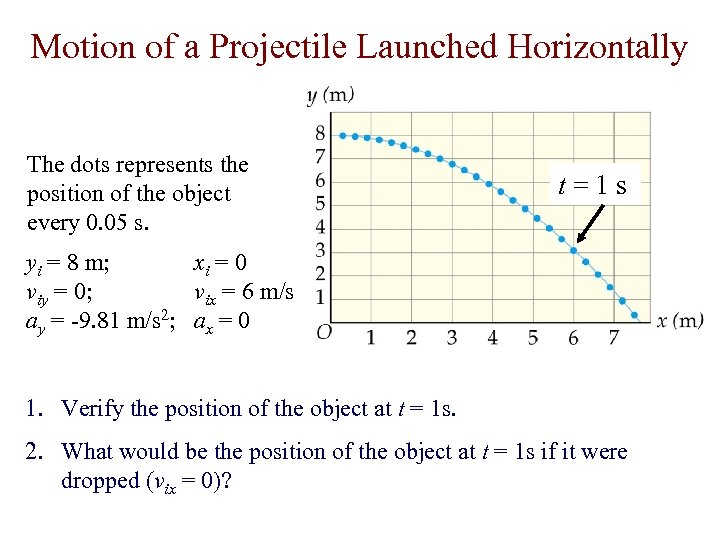Motion of a Projectile Launched Horizontally The dots represents the position of the object every 0. 05 s. t=1 s yi = 8 m; xi = 0 viy = 0; vix = 6 m/s ay = -9. 81 m/s 2; ax = 0 1. Verify the position of the object at t = 1 s. 2. What would be the position of the object at t = 1 s if it were dropped (vix = 0)?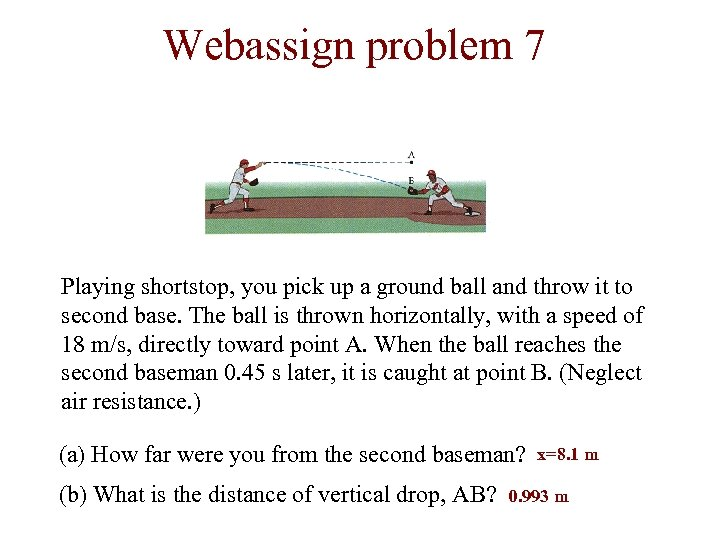Webassign problem 7 Playing shortstop, you pick up a ground ball and throw it to second base. The ball is thrown horizontally, with a speed of 18 m/s, directly toward point A. When the ball reaches the second baseman 0. 45 s later, it is caught at point B. (Neglect air resistance. ) (a) How far were you from the second baseman? (b) What is the distance of vertical drop, AB? x=8. 1 m 0. 993 m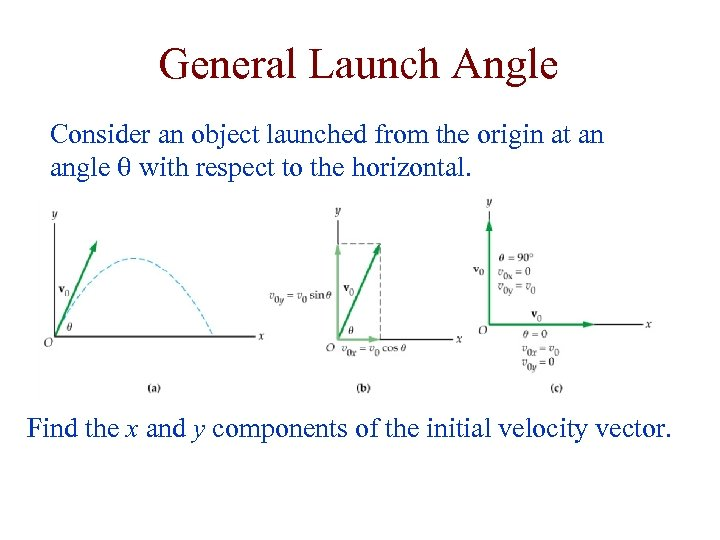General Launch Angle Consider an object launched from the origin at an angle q with respect to the horizontal. Find the x and y components of the initial velocity vector.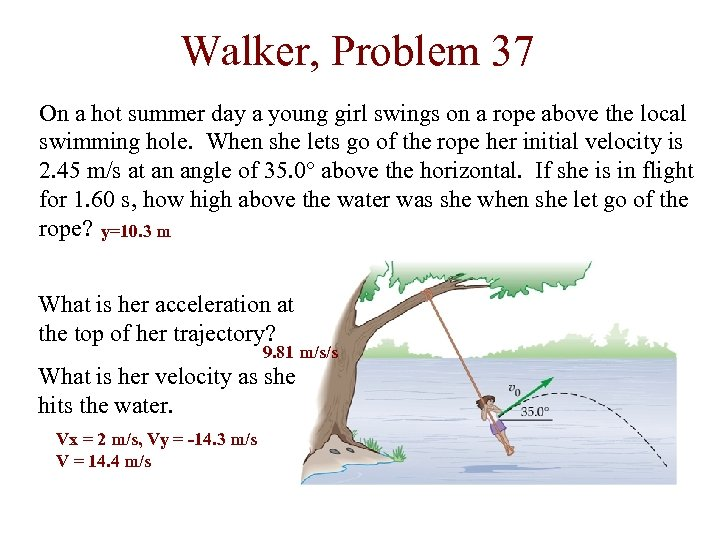Walker, Problem 37 On a hot summer day a young girl swings on a rope above the local swimming hole. When she lets go of the rope her initial velocity is 2. 45 m/s at an angle of 35. 0° above the horizontal. If she is in flight for 1. 60 s, how high above the water was she when she let go of the rope? y=10. 3 m What is her acceleration at the top of her trajectory? 9. 81 m/s/s What is her velocity as she hits the water. Vx = 2 m/s, Vy = -14. 3 m/s V = 14. 4 m/s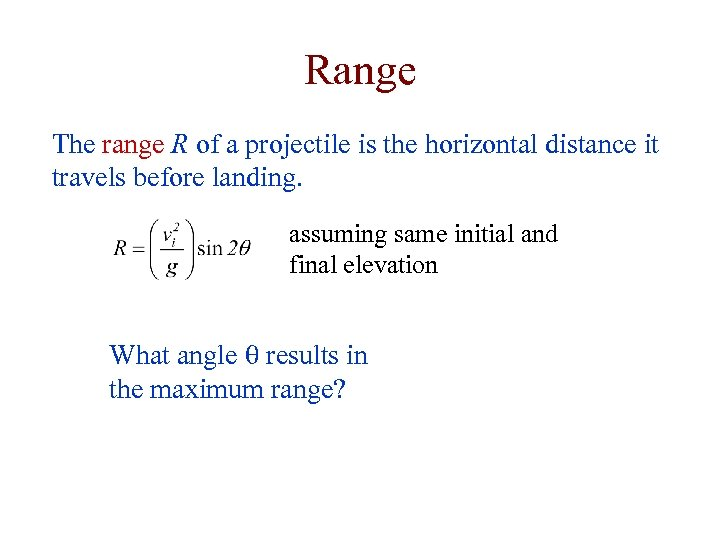Range The range R of a projectile is the horizontal distance it travels before landing. assuming same initial and final elevation What angle q results in the maximum range?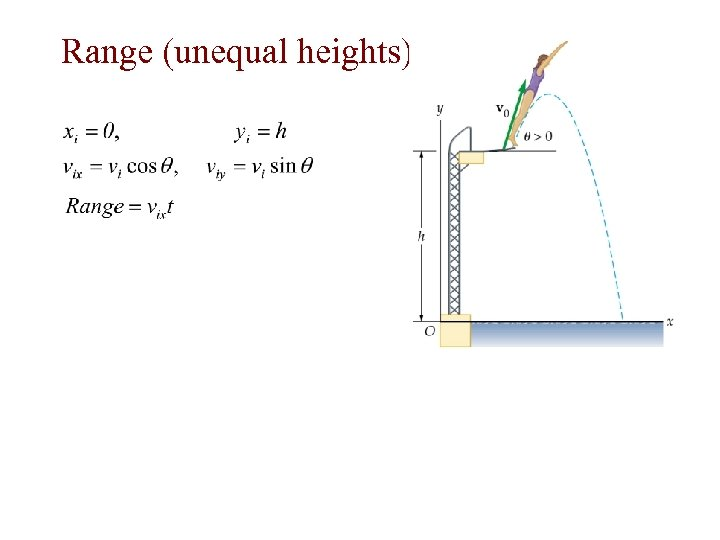Range (unequal heights)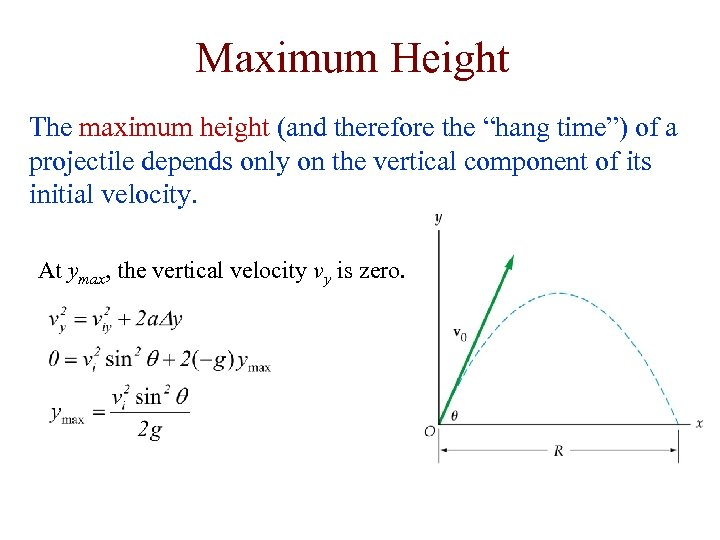Maximum Height The maximum height (and therefore the “hang time”) of a projectile depends only on the vertical component of its initial velocity. At ymax, the vertical velocity vy is zero.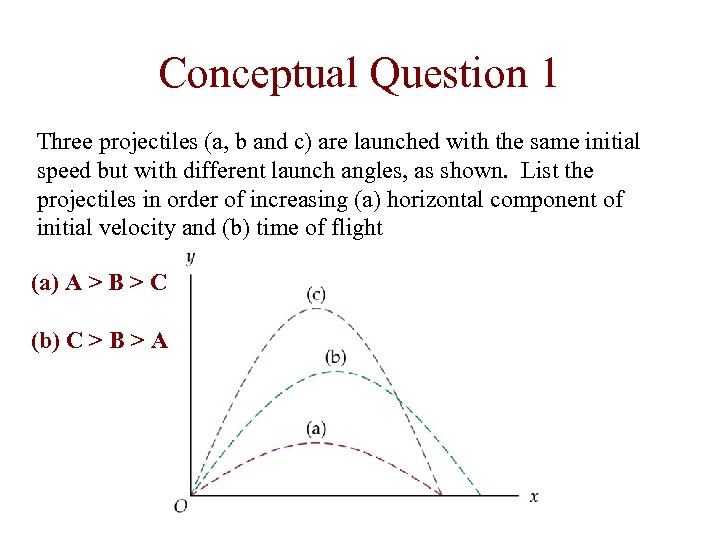Conceptual Question 1 Three projectiles (a, b and c) are launched with the same initial speed but with different launch angles, as shown. List the projectiles in order of increasing (a) horizontal component of initial velocity and (b) time of flight (a) A > B > C (b) C > B > A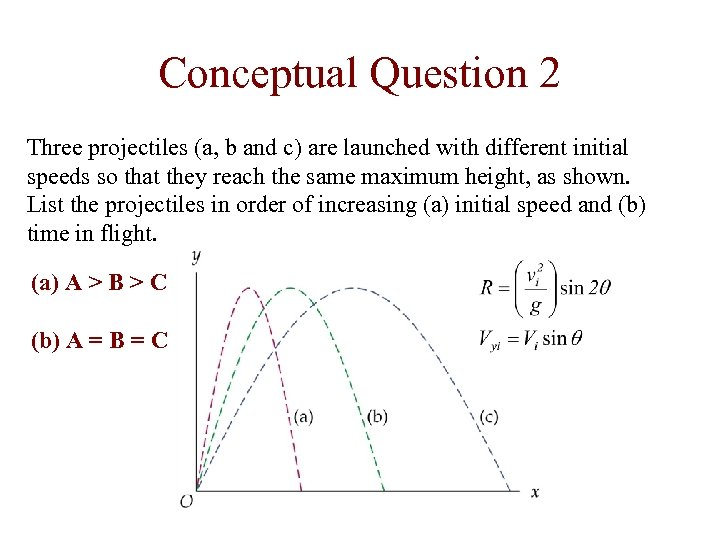Conceptual Question 2 Three projectiles (a, b and c) are launched with different initial speeds so that they reach the same maximum height, as shown. List the projectiles in order of increasing (a) initial speed and (b) time in flight. (a) A > B > C (b) A = B = C